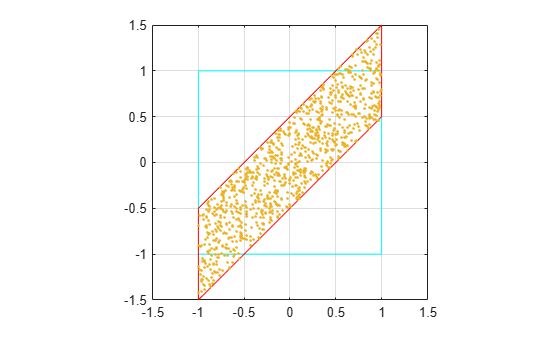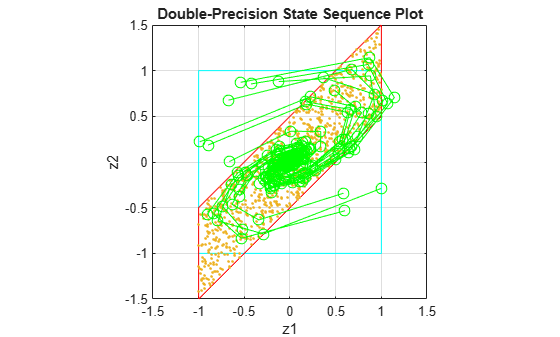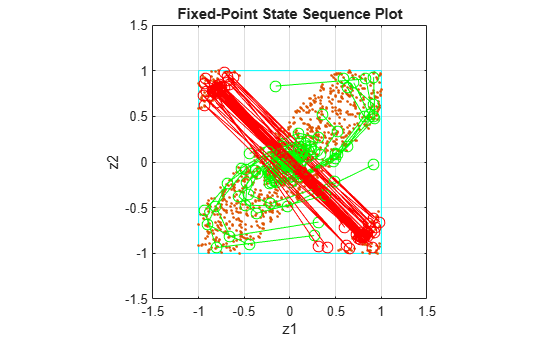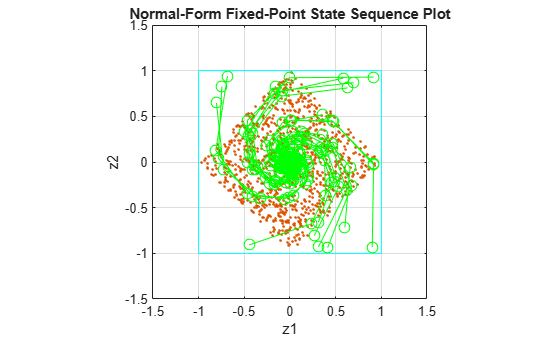# Detect Limit Cycles in Fixed-Point State-Space Systems

This example shows how to analyze a fixed-point state-space system to detect limit cycles.

The example focuses on detecting large scale limit cycles due to overflow with zero inputs and highlights the conditions that are sufficient to prevent such oscillations.

### Select a State-Space Representation of the System

See that the system is stable by observing that the eigenvalues of the state-transition matrix `A` have magnitudes less than `1`.

```A = [0 1; -.5 1];B = [0; 1]; C = [1 0]; D = 0; eig(A)```
```ans = 2×1 complex 0.5000 + 0.5000i 0.5000 - 0.5000i ```

### Filter Implementation

The function `fisisostatespacefilter` implements a single-input single-output state-space filter.

`type('fisisostatespacefilter.m')`
```function [y,z] = fisisostatespacefilter(A,B,C,D,x,z) %FISISOSTATESPACEFILTER Single-input, single-output statespace filter % [Y,Zf] = FISISOSTATESPACEFILTER(A,B,C,D,X,Zi) filters data X with % initial conditions Zi with the state-space filter defined by matrices % A, B, C, D. Output Y and final conditions Zf are returned. % Copyright 2004-2011 The MathWorks, Inc. y = x; z(:,2:length(x)+1) = 0; for k=1:length(x) y(k) = C*z(:,k) + D*x(k); z(:,k+1) = A*z(:,k) + B*x(k); end ```

### Floating-Point Filter

Create a floating-point filter and observe the trajectory of the states.

First, choose random states within the unit square and observe where they are projected after one step of being multiplied by the state-transition matrix A.

```rng('default'); clf x1 = [-1 1 1 -1 -1]; y1 = [-1 -1 1 1 -1]; plot(x1,y1,'c') axis([-1.5 1.5 -1.5 1.5]); axis square; grid; hold on```

Plot the projection of the square.

```p = A*[x1;y1]; plot(p(1,:),p(2,:),'r') r = 2*rand(2,1000)-1; pr = A*r; plot(pr(1,:),pr(2,:),'.')```### Follow Random Initial States Through Time

Drive the filter with a random initial state, normalized to be inside the unit square, with the input all zero, and run the filter.

```x = zeros(10,1); zi = [0;0]; q = quantizer([16 15]); for k=1:20 y = x; zi(:) = randquant(q,size(A,1),1); [y,zf] = fisisostatespacefilter(A,B,C,D,x,zi); plot(zf(1,:), zf(2,:),'go-','markersize',8); end title('Double-Precision State Sequence Plot'); xlabel('z1'); ylabel('z2')```Some of the states wander outside the unit square and eventually wind down to the zero state at the origin, `z = [0;0]`.

### State Trajectory

Because the eigenvalues are less than one in magnitude, the system is stable and all initial states wind down to the origin with zero input. However, the eigenvalues don't tell the whole story about the trajectory of the states, as in this example, where the states were projected outward first before they start to contract.

The singular values of A give us a better indication of the overall state trajectory.

`svd(A)`
```ans = 2×1 1.4604 0.3424 ```

The largest singular value is about 1.46, which indicates that states aligned with the corresponding singular vector will be projected away from the origin.

### Create Fixed-Point Filter

Create a fixed-point filter and check for limit cycles.

The MATLAB® code for the filter remains the same. It becomes a fixed-point filter when it is driven with fixed-point inputs. For the sake of illustrating overflow oscillation, choose product and sum data types that will overflow.

```rng('default'); F = fimath('OverflowAction','Wrap',... 'ProductMode','SpecifyPrecision',... 'ProductWordLength',16,'ProductFractionLength',15,... 'SumMode','SpecifyPrecision',... 'SumWordLength',16,'SumFractionLength',15); A = fi(A,'fimath',F)```
```A = 0 1.0000 -0.5000 1.0000 DataTypeMode: Fixed-point: binary point scaling Signedness: Signed WordLength: 16 FractionLength: 14 RoundingMethod: Nearest OverflowAction: Wrap ProductMode: SpecifyPrecision ProductWordLength: 16 ProductFractionLength: 15 SumMode: SpecifyPrecision SumWordLength: 16 SumFractionLength: 15 CastBeforeSum: true ```
`B = fi(B,'fimath',F)`
```B = 0 1 DataTypeMode: Fixed-point: binary point scaling Signedness: Signed WordLength: 16 FractionLength: 14 RoundingMethod: Nearest OverflowAction: Wrap ProductMode: SpecifyPrecision ProductWordLength: 16 ProductFractionLength: 15 SumMode: SpecifyPrecision SumWordLength: 16 SumFractionLength: 15 CastBeforeSum: true ```
`C = fi(C,'fimath',F)`
```C = 1 0 DataTypeMode: Fixed-point: binary point scaling Signedness: Signed WordLength: 16 FractionLength: 14 RoundingMethod: Nearest OverflowAction: Wrap ProductMode: SpecifyPrecision ProductWordLength: 16 ProductFractionLength: 15 SumMode: SpecifyPrecision SumWordLength: 16 SumFractionLength: 15 CastBeforeSum: true ```
`D = fi(D,'fimath',F)`
```D = 0 DataTypeMode: Fixed-point: binary point scaling Signedness: Signed WordLength: 16 FractionLength: 15 RoundingMethod: Nearest OverflowAction: Wrap ProductMode: SpecifyPrecision ProductWordLength: 16 ProductFractionLength: 15 SumMode: SpecifyPrecision SumWordLength: 16 SumFractionLength: 15 CastBeforeSum: true ```

### Plot Projection of Square in Fixed-Point

Choose random states within the unit square and observe where they are projected after one step of being multiplied by the state-transition matrix `A`. This time the matrix A is fixed-point.

```clf r = 2*rand(2,1000)-1; pr = A*r; plot([-1 1 1 -1 -1],[-1 -1 1 1 -1],'c') axis([-1.5 1.5 -1.5 1.5]); axis square; grid; hold on plot(pr(1,:),pr(2,:),'.')```The triangles that projected out of the square in floating-point are now wrapped back into the interior of the square.

### Execute Fixed-Point Filter

Drive the filter with fixed-point data types.

```x = fi(zeros(10,1),1,16,15,'fimath',F); zi = fi([0;0],1,16,15,'fimath',F); q = assignmentquantizer(zi); e = double(eps(zi)); rng('default'); for k=1:20 y = x; zi(:) = randquant(q,size(A,1),1); [y,zf] = fisisostatespacefilter(A,B,C,D,x,zi); if abs(double(zf(end)))>0.5, c='ro-'; else, c='go-'; end plot(zf(1,:), zf(2,:),c,'markersize',8); end title('Fixed-Point State Sequence Plot'); xlabel('z1'); ylabel('z2')```Trying this for other randomly chosen initial states illustrates that once a state enters one of the triangular regions, then it is projected into the other triangular region, and back and forth, and never escapes.

### Sufficient Conditions for Preventing Overflow Limit Cycles

There are two sufficient conditions to prevent overflow limit cycles in a system:

• The system is stable: `abs(eig(A))<1`

• The matrix A is normal: `A'*A = A*A'`

For the current representation, the second condition does not hold.

### Apply Similarity Transform to Create Normal `A`

Apply a similarity transformation to the original system to create a normal state-transition matrix `A2`.

```T = [-2 0;-1 1]; Tinv = [-.5 0;-.5 1]; A2 = Tinv*A*T; B2 = Tinv*B; C2 = C*T; D2 = D;```

Similarity transformations preserve eigenvalues. The system transfer function of the transformed system remains same as before. However, the transformed state transformation matrix `A2` is normal.

### Check for Limit Cycles on Transformed System

Plot the projection of the square of the normal-form system.

```clf r = 2*rand(2,1000)-1; pr = A2*r; plot([-1 1 1 -1 -1],[-1 -1 1 1 -1],'c') axis([-1.5 1.5 -1.5 1.5]); axis square; grid; hold on plot(pr(1,:),pr(2,:),'.')```

Now the projection of random initial states inside the unit square all contract uniformly. This is the result of the state transition matrix `A2` being normal. The states are also rotated by 90 degrees counterclockwise.

Plot the state sequences again for the same initial states as before. Observe that the outputs now spiral towards the origin.

```x = fi(zeros(10,1),1,16,15,'fimath',F); zi = fi([0;0],1,16,15,'fimath',F); q = assignmentquantizer(zi); e = double(eps(zi)); rng('default'); for k=1:20 y = x; zi(:) = randquant(q,size(A,1),1); [y,zf] = fisisostatespacefilter(A2,B2,C2,D2,x,zi); if abs(double(zf(end)))>0.5, c='ro-'; else, c='go-'; end plot(zf(1,:), zf(2,:),c,'markersize',8); end title('Normal-Form Fixed-Point State Sequence Plot'); xlabel('z1'); ylabel('z2')```Trying this for other randomly chosen initial states illustrates that there is no region from which the filter is unable to recover.

`%#ok<*NASGU,*NOPTS>`

### References

 Richard A. Roberts and Clifford T. Mullis, "Digital Signal Processing", Addison-Wesley, Reading, Massachusetts, 1987, ISBN 0-201-16350-0, Section 9.3.

 S. K. Mitra, "Digital Signal Processing: A Computer Based Approach", McGraw-Hill, New York, 1998, ISBN 0-07-042953-7.